# chapter 16 Recursion Another Control Mechanism a function

• Slides: 49
Download presentationchapter 16 Recursion: Another Control Mechanism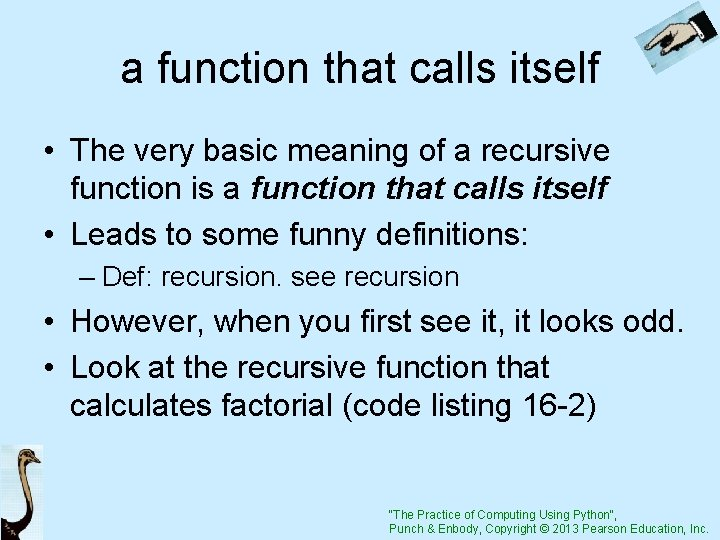a function that calls itself • The very basic meaning of a recursive function is a function that calls itself • Leads to some funny definitions: – Def: recursion. see recursion • However, when you first see it, it looks odd. • Look at the recursive function that calculates factorial (code listing 16 -2) "The Practice of Computing Using Python", Punch & Enbody, Copyright © 2013 Pearson Education, Inc.It doesn’t do anything! • This is a common complaint when one first sees a recursive function. What exactly is it doing? It doesn’t seem to do anything! • Our goal is to understand what it means to write a recursive function from a programmers and computers view. "The Practice of Computing Using Python", Punch & Enbody, Copyright © 2013 Pearson Education, Inc.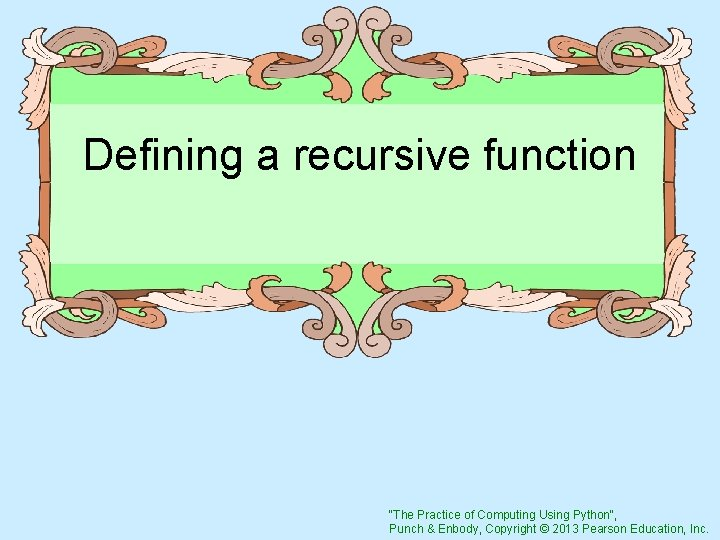Defining a recursive function "The Practice of Computing Using Python", Punch & Enbody, Copyright © 2013 Pearson Education, Inc.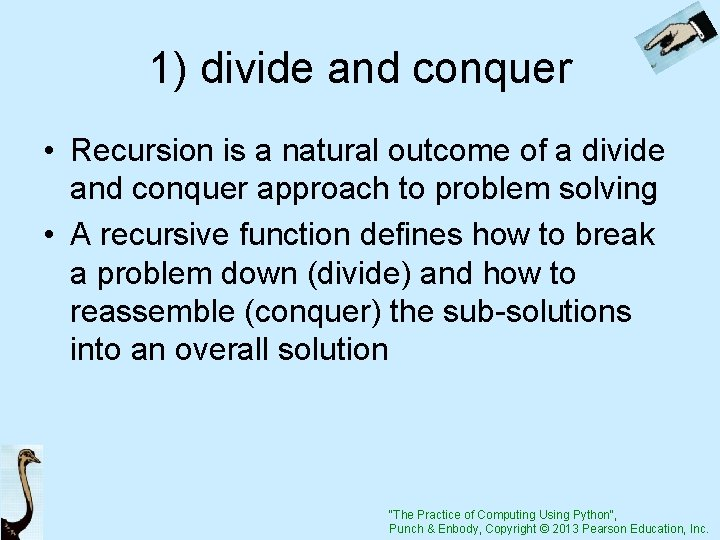1) divide and conquer • Recursion is a natural outcome of a divide and conquer approach to problem solving • A recursive function defines how to break a problem down (divide) and how to reassemble (conquer) the sub-solutions into an overall solution "The Practice of Computing Using Python", Punch & Enbody, Copyright © 2013 Pearson Education, Inc.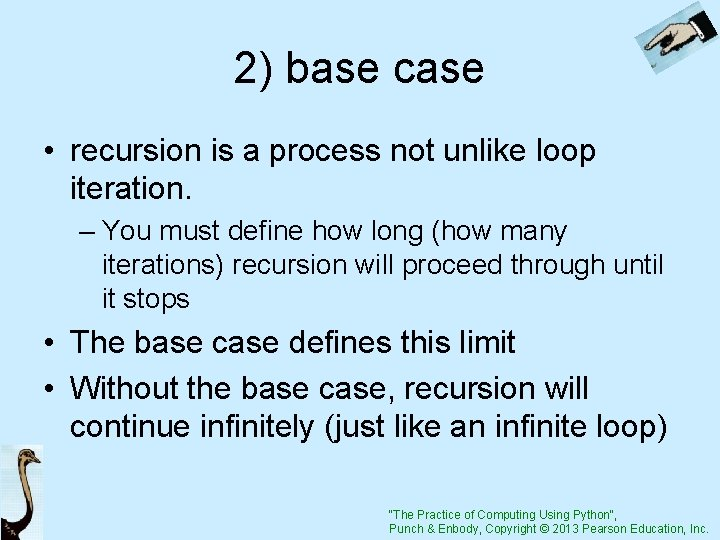2) base case • recursion is a process not unlike loop iteration. – You must define how long (how many iterations) recursion will proceed through until it stops • The base case defines this limit • Without the base case, recursion will continue infinitely (just like an infinite loop) "The Practice of Computing Using Python", Punch & Enbody, Copyright © 2013 Pearson Education, Inc.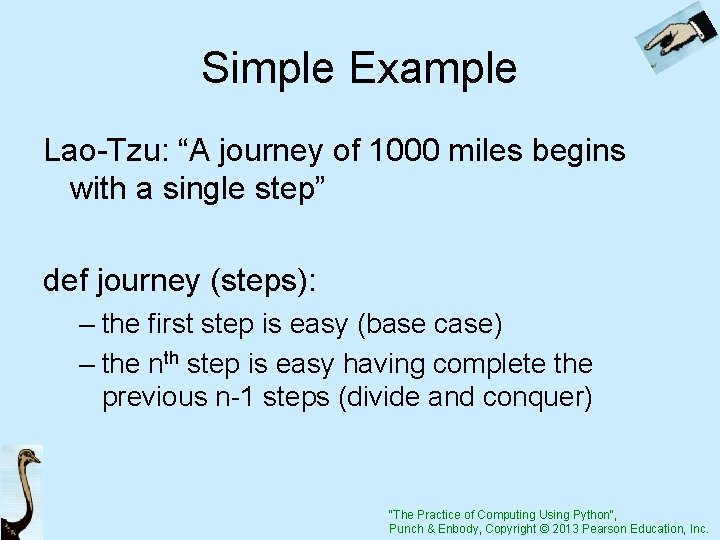Simple Example Lao-Tzu: “A journey of 1000 miles begins with a single step” def journey (steps): – the first step is easy (base case) – the nth step is easy having complete the previous n-1 steps (divide and conquer) "The Practice of Computing Using Python", Punch & Enbody, Copyright © 2013 Pearson Education, Inc.Code Listing 16 -1 "The Practice of Computing Using Python", Punch & Enbody, Copyright © 2013 Pearson Education, Inc."The Practice of Computing Using Python", Punch & Enbody, Copyright © 2013 Pearson Education, Inc.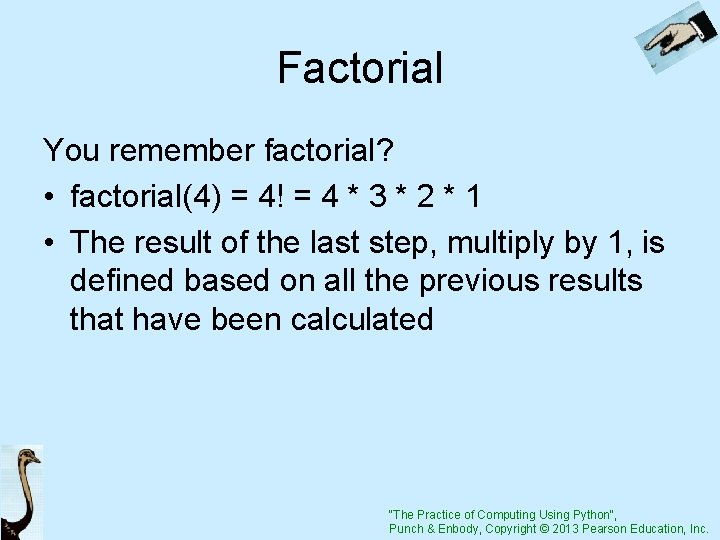Factorial You remember factorial? • factorial(4) = 4! = 4 * 3 * 2 * 1 • The result of the last step, multiply by 1, is defined based on all the previous results that have been calculated "The Practice of Computing Using Python", Punch & Enbody, Copyright © 2013 Pearson Education, Inc.Code Listing 16 -2 "The Practice of Computing Using Python", Punch & Enbody, Copyright © 2013 Pearson Education, Inc."The Practice of Computing Using Python", Punch & Enbody, Copyright © 2013 Pearson Education, Inc.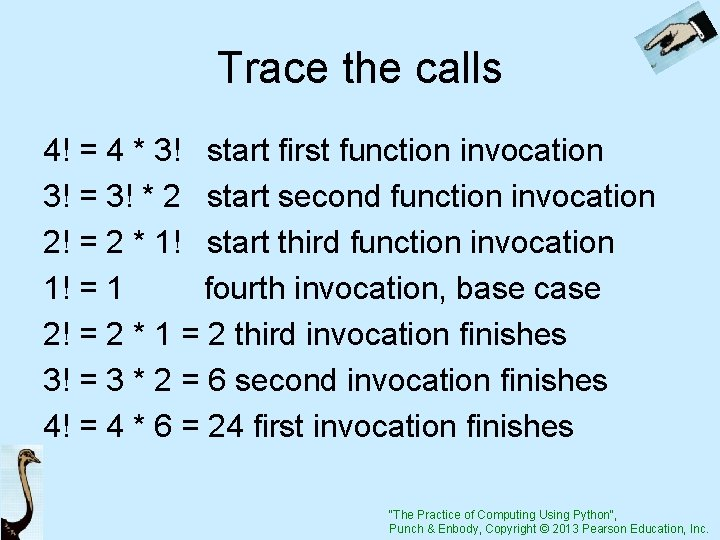Trace the calls 4! = 4 * 3! start first function invocation 3! = 3! * 2 start second function invocation 2! = 2 * 1! start third function invocation 1! = 1 fourth invocation, base case 2! = 2 * 1 = 2 third invocation finishes 3! = 3 * 2 = 6 second invocation finishes 4! = 4 * 6 = 24 first invocation finishes "The Practice of Computing Using Python", Punch & Enbody, Copyright © 2013 Pearson Education, Inc.Another: Fibonacci • Depends on the Fibonacci results for the two previous values in the sequence • The base values are Fibo(0) == 1 and Fibo(1) == 1 Fibo (x) = fibo(x-1) + fibo(x-2) "The Practice of Computing Using Python", Punch & Enbody, Copyright © 2013 Pearson Education, Inc.Code Listing 16 -3 "The Practice of Computing Using Python", Punch & Enbody, Copyright © 2013 Pearson Education, Inc.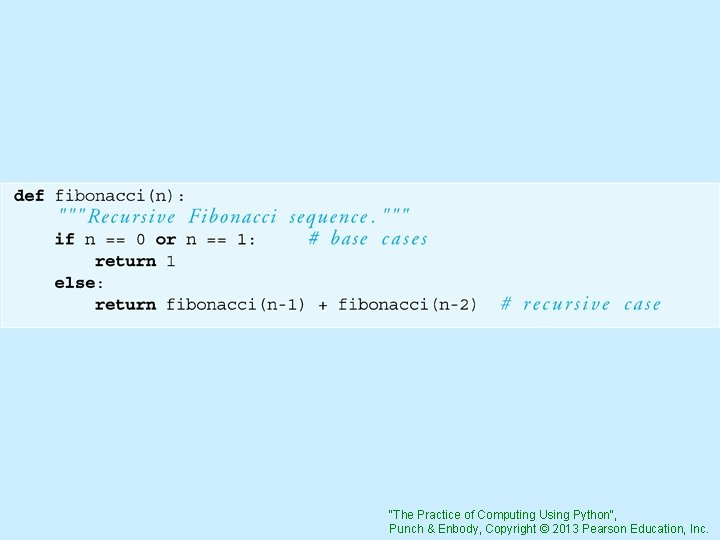"The Practice of Computing Using Python", Punch & Enbody, Copyright © 2013 Pearson Education, Inc.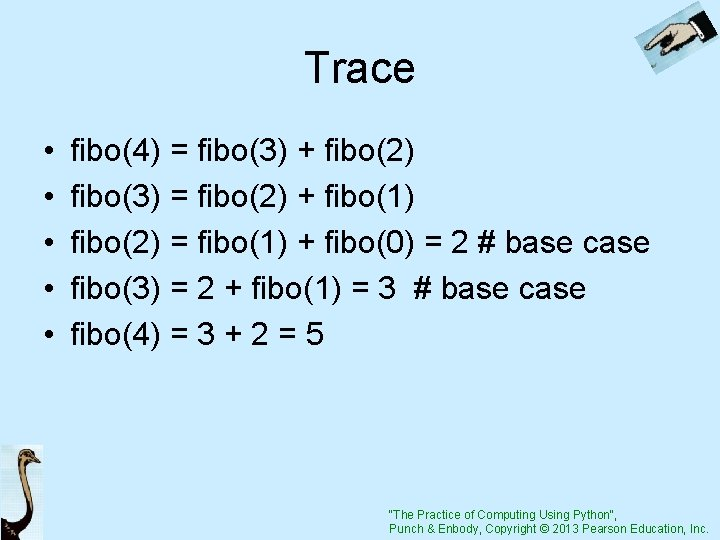Trace • • • fibo(4) = fibo(3) + fibo(2) fibo(3) = fibo(2) + fibo(1) fibo(2) = fibo(1) + fibo(0) = 2 # base case fibo(3) = 2 + fibo(1) = 3 # base case fibo(4) = 3 + 2 = 5 "The Practice of Computing Using Python", Punch & Enbody, Copyright © 2013 Pearson Education, Inc.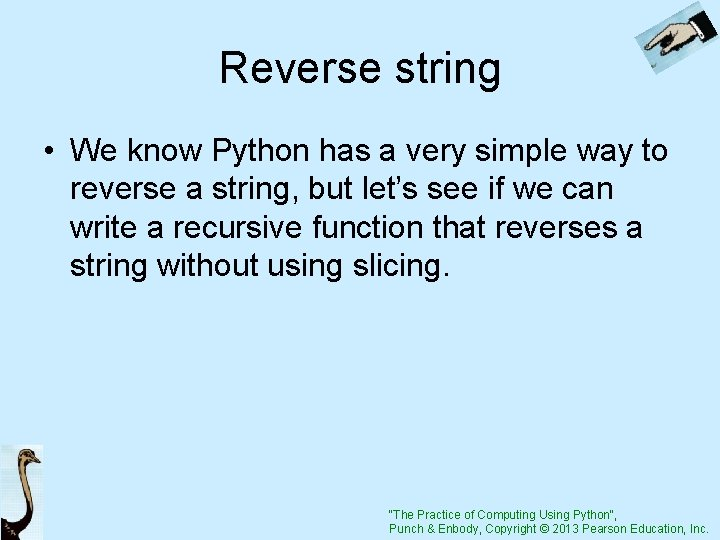Reverse string • We know Python has a very simple way to reverse a string, but let’s see if we can write a recursive function that reverses a string without using slicing. "The Practice of Computing Using Python", Punch & Enbody, Copyright © 2013 Pearson Education, Inc.Code Listing 16 -4 Template for reversal "The Practice of Computing Using Python", Punch & Enbody, Copyright © 2013 Pearson Education, Inc.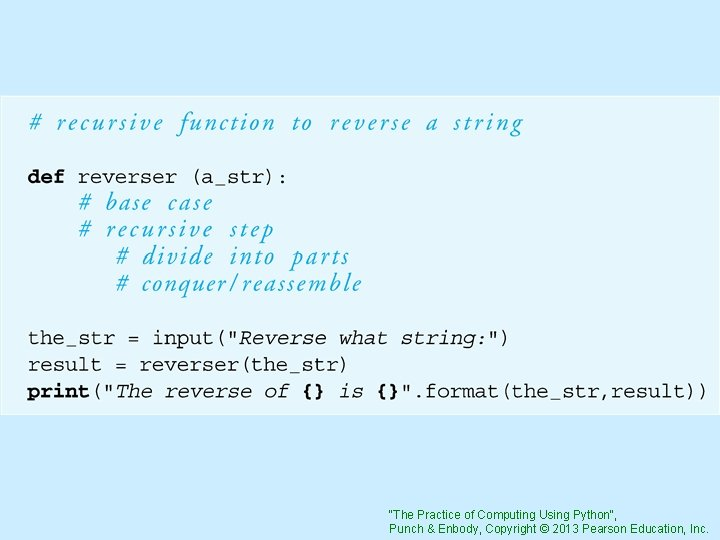"The Practice of Computing Using Python", Punch & Enbody, Copyright © 2013 Pearson Education, Inc.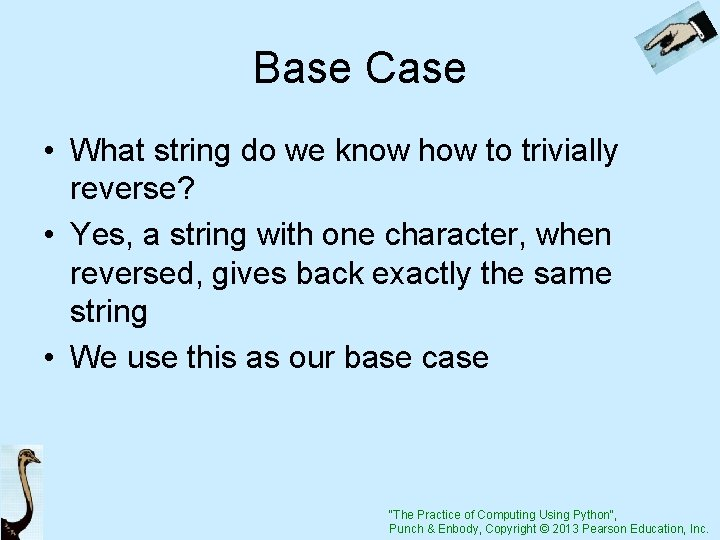Base Case • What string do we know how to trivially reverse? • Yes, a string with one character, when reversed, gives back exactly the same string • We use this as our base case "The Practice of Computing Using Python", Punch & Enbody, Copyright © 2013 Pearson Education, Inc.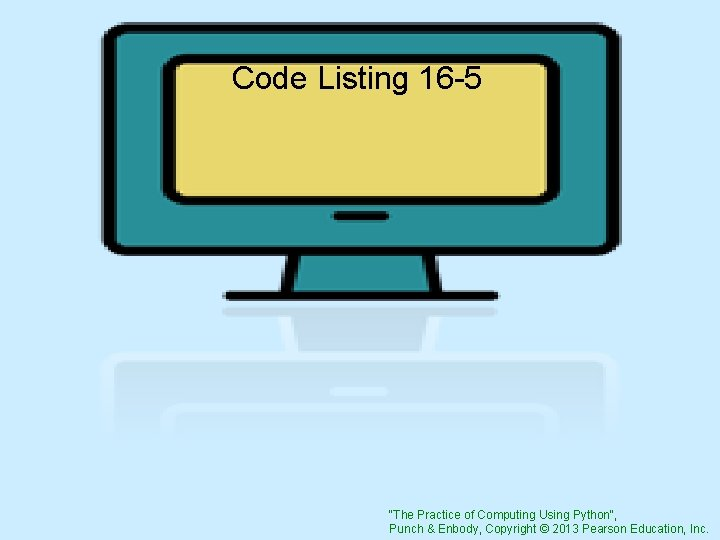Code Listing 16 -5 "The Practice of Computing Using Python", Punch & Enbody, Copyright © 2013 Pearson Education, Inc.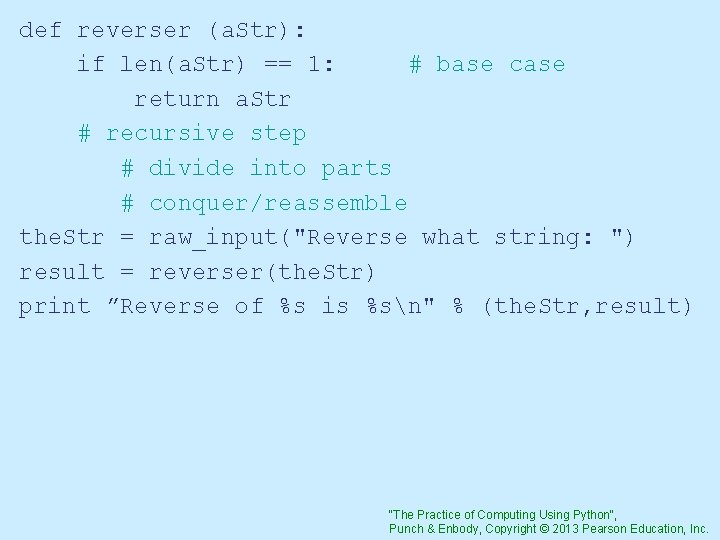def reverser (a. Str): if len(a. Str) == 1: # base case return a. Str # recursive step # divide into parts # conquer/reassemble the. Str = raw_input("Reverse what string: ") result = reverser(the. Str) print ”Reverse of %s is %sn" % (the. Str, result) "The Practice of Computing Using Python", Punch & Enbody, Copyright © 2013 Pearson Education, Inc.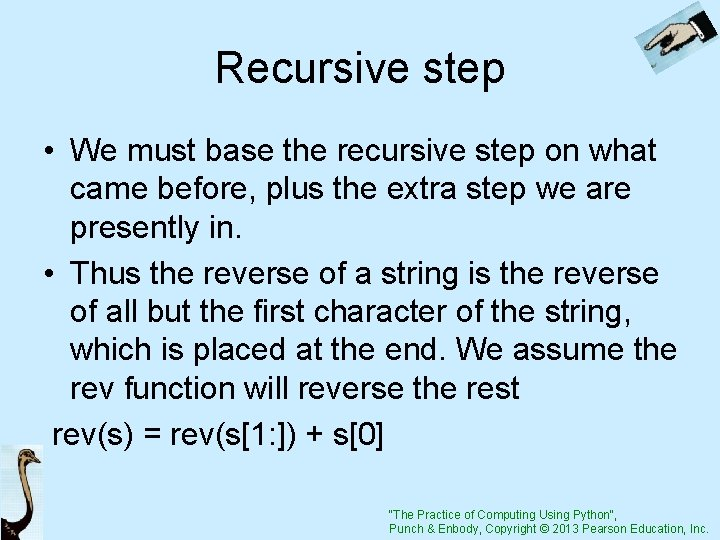Recursive step • We must base the recursive step on what came before, plus the extra step we are presently in. • Thus the reverse of a string is the reverse of all but the first character of the string, which is placed at the end. We assume the rev function will reverse the rest rev(s) = rev(s[1: ]) + s "The Practice of Computing Using Python", Punch & Enbody, Copyright © 2013 Pearson Education, Inc.Code Listing 16 -6 "The Practice of Computing Using Python", Punch & Enbody, Copyright © 2013 Pearson Education, Inc.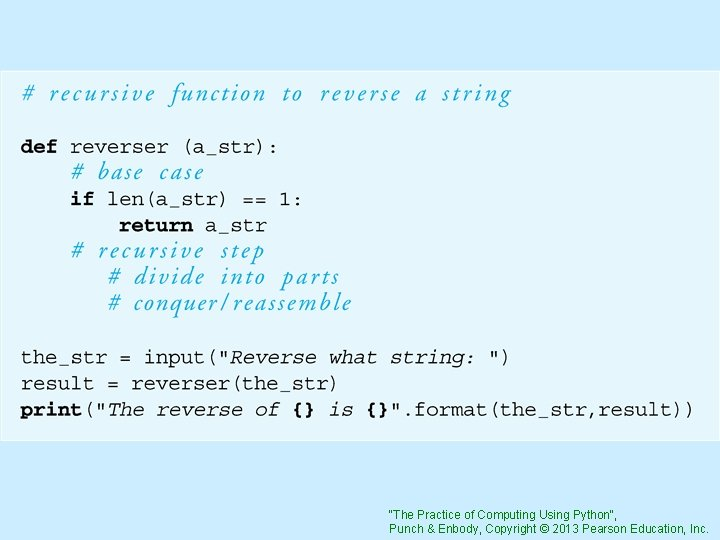"The Practice of Computing Using Python", Punch & Enbody, Copyright © 2013 Pearson Education, Inc.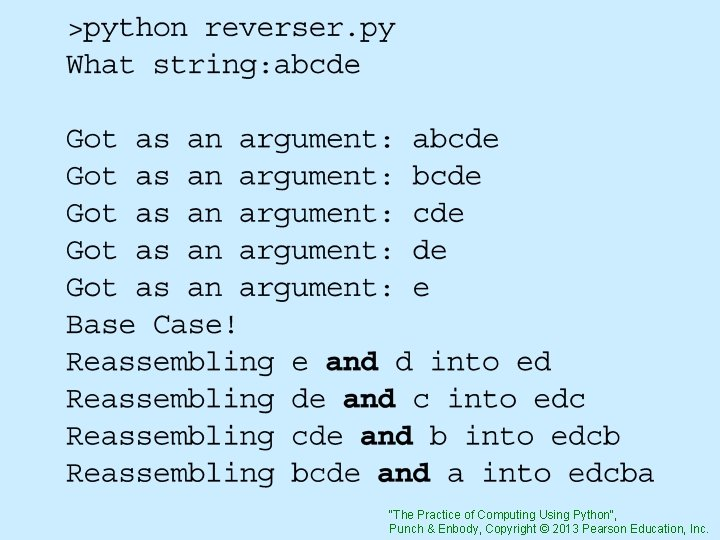"The Practice of Computing Using Python", Punch & Enbody, Copyright © 2013 Pearson Education, Inc.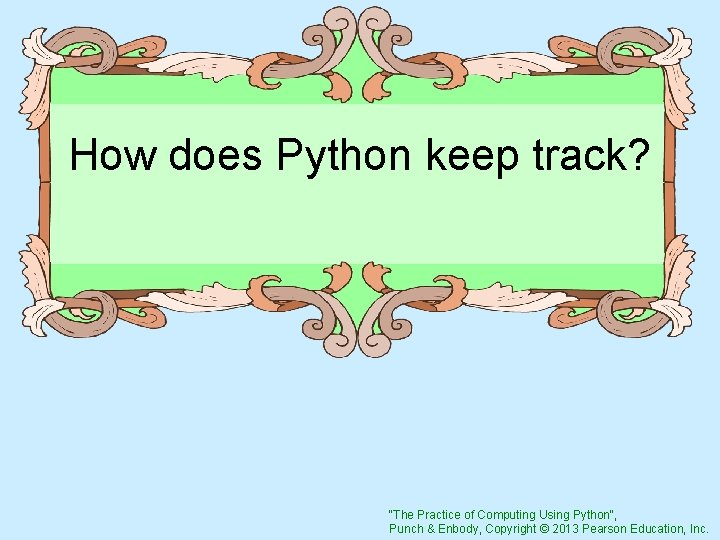How does Python keep track? "The Practice of Computing Using Python", Punch & Enbody, Copyright © 2013 Pearson Education, Inc.The Stack • A Stack is a data structure, like a List or a Dictionary, but with a few different characteristics • A Stack is a sequence • A stack only allows access to one end of its data, the top of the stack "The Practice of Computing Using Python", Punch & Enbody, Copyright © 2013 Pearson Education, Inc."The Practice of Computing Using Python", Punch & Enbody, Copyright © 2013 Pearson Education, Inc.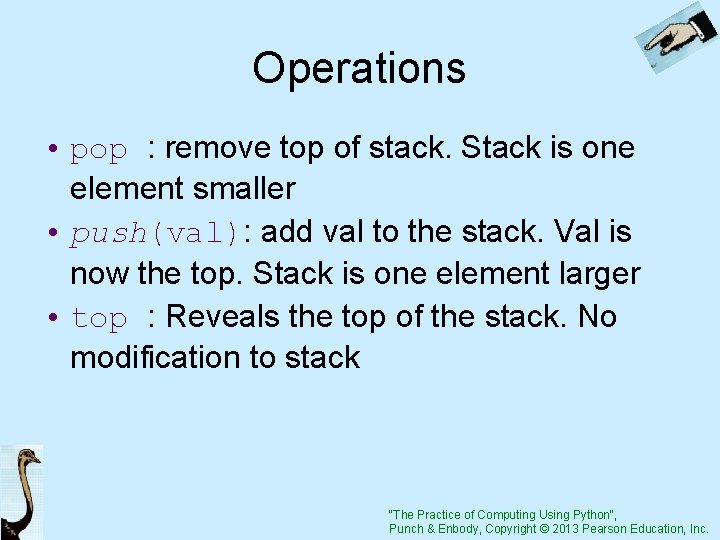Operations • pop : remove top of stack. Stack is one element smaller • push(val): add val to the stack. Val is now the top. Stack is one element larger • top : Reveals the top of the stack. No modification to stack "The Practice of Computing Using Python", Punch & Enbody, Copyright © 2013 Pearson Education, Inc.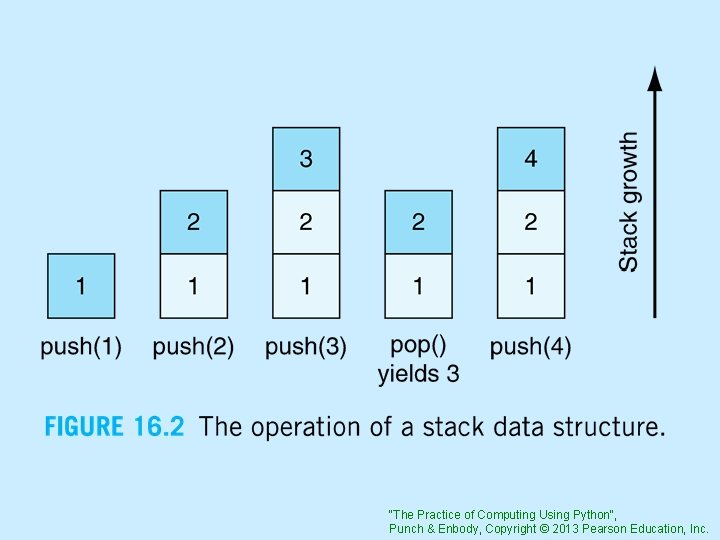"The Practice of Computing Using Python", Punch & Enbody, Copyright © 2013 Pearson Education, Inc.Stack of function calls Python maintains a stack of function calls. • If a function calls another function recursively, the new function is pushed onto the calling stack and the previous function waits • The top is always the active function • When a pop occurs, the function below becomes active "The Practice of Computing Using Python", Punch & Enbody, Copyright © 2013 Pearson Education, Inc.Code Listing 16 -7 "The Practice of Computing Using Python", Punch & Enbody, Copyright © 2013 Pearson Education, Inc.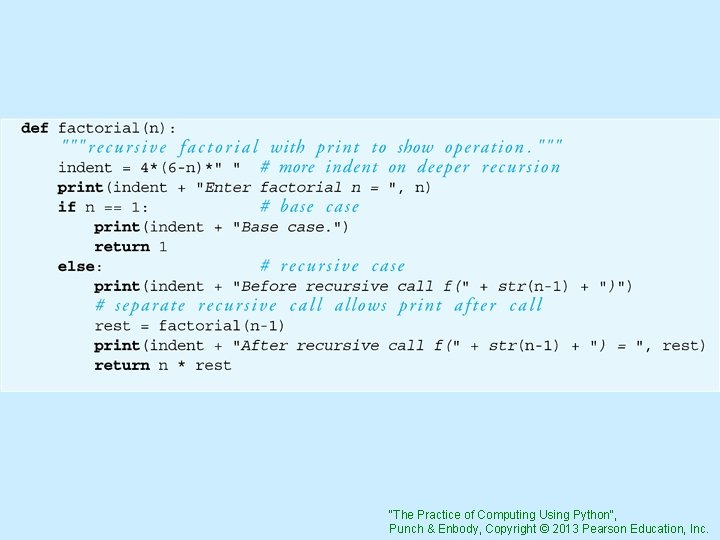"The Practice of Computing Using Python", Punch & Enbody, Copyright © 2013 Pearson Education, Inc.>>> factorial(4) Enter factorial n = 4 Before recursive call f(3) Enter factorial n = 3 Before recursive call f(2) Enter factorial n = 2 Before recursive call f(1) Enter factorial n = 1 Base case. After recursive call f(1) = After recursive call f(2) = 2 After recursive call f(3) = 6 24 1 "The Practice of Computing Using Python", Punch & Enbody, Copyright © 2013 Pearson Education, Inc.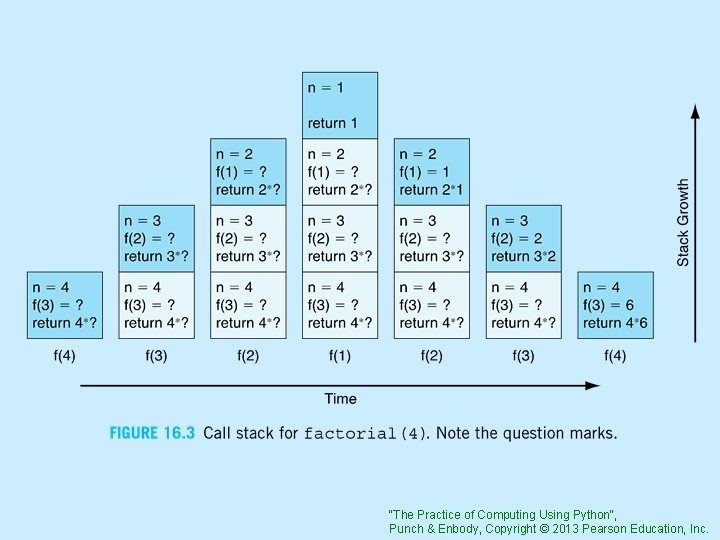"The Practice of Computing Using Python", Punch & Enbody, Copyright © 2013 Pearson Education, Inc.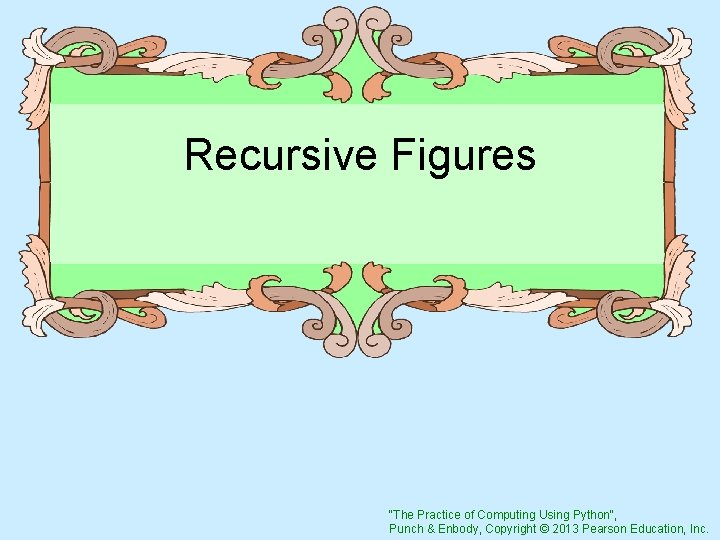Recursive Figures "The Practice of Computing Using Python", Punch & Enbody, Copyright © 2013 Pearson Education, Inc.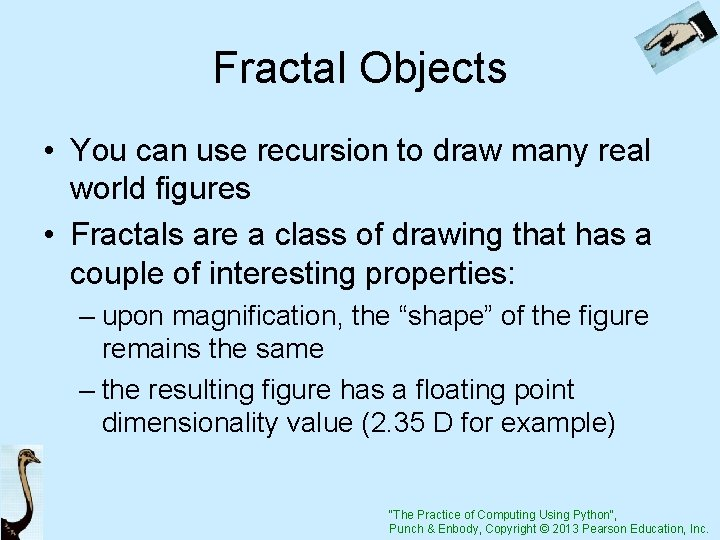Fractal Objects • You can use recursion to draw many real world figures • Fractals are a class of drawing that has a couple of interesting properties: – upon magnification, the “shape” of the figure remains the same – the resulting figure has a floating point dimensionality value (2. 35 D for example) "The Practice of Computing Using Python", Punch & Enbody, Copyright © 2013 Pearson Education, Inc.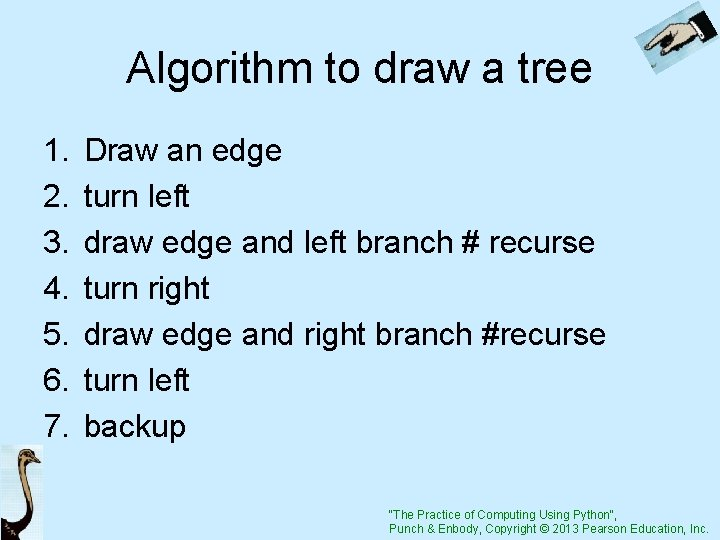Algorithm to draw a tree 1. 2. 3. 4. 5. 6. 7. Draw an edge turn left draw edge and left branch # recurse turn right draw edge and right branch #recurse turn left backup "The Practice of Computing Using Python", Punch & Enbody, Copyright © 2013 Pearson Education, Inc.Code Listing 16 -8 "The Practice of Computing Using Python", Punch & Enbody, Copyright © 2013 Pearson Education, Inc.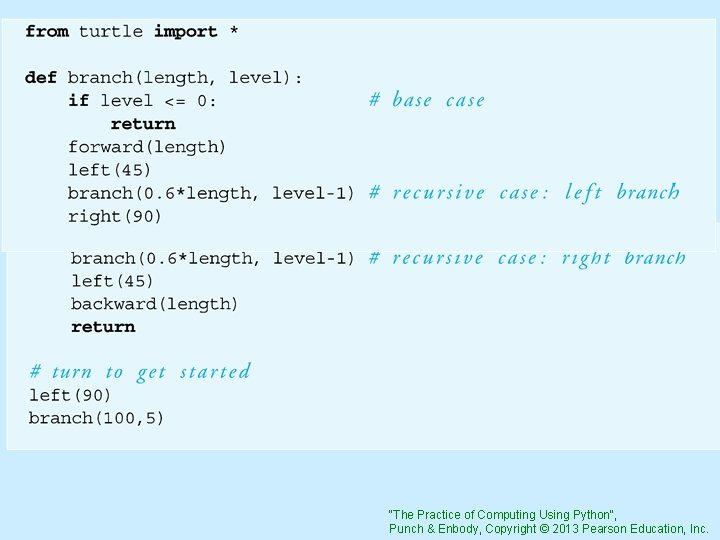"The Practice of Computing Using Python", Punch & Enbody, Copyright © 2013 Pearson Education, Inc.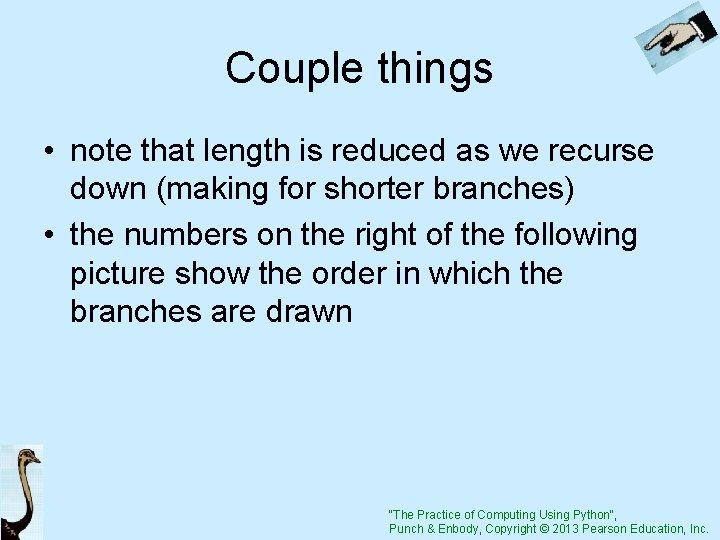Couple things • note that length is reduced as we recurse down (making for shorter branches) • the numbers on the right of the following picture show the order in which the branches are drawn "The Practice of Computing Using Python", Punch & Enbody, Copyright © 2013 Pearson Education, Inc.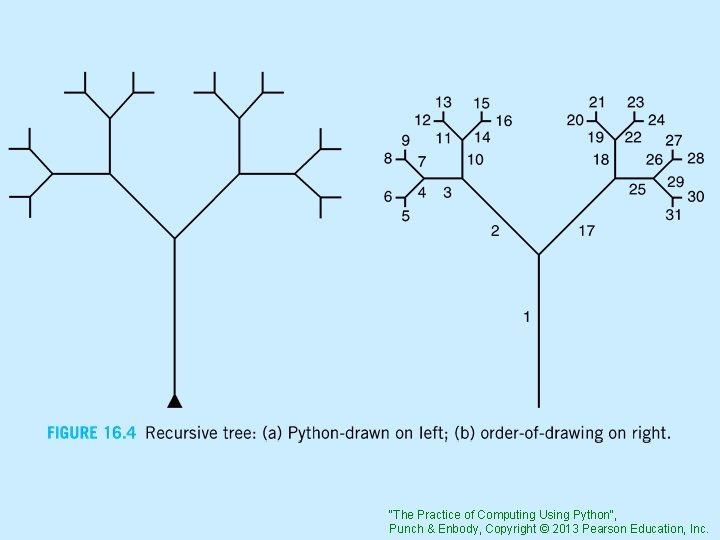"The Practice of Computing Using Python", Punch & Enbody, Copyright © 2013 Pearson Education, Inc.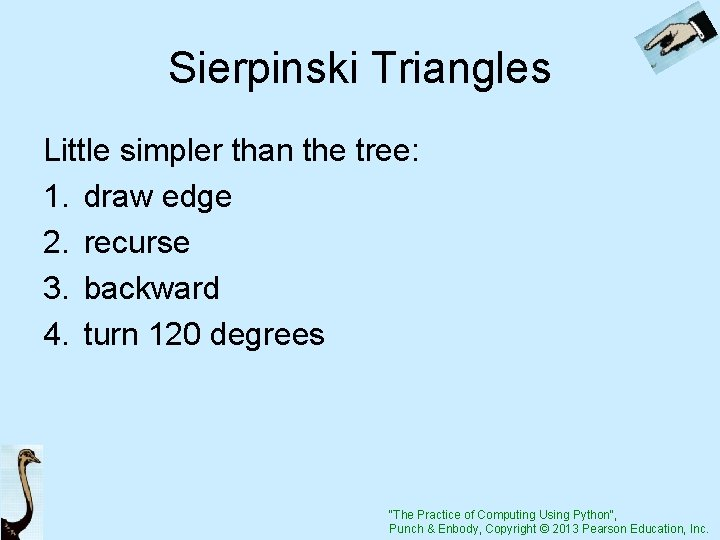Sierpinski Triangles Little simpler than the tree: 1. draw edge 2. recurse 3. backward 4. turn 120 degrees "The Practice of Computing Using Python", Punch & Enbody, Copyright © 2013 Pearson Education, Inc.Code Listing 16 -9 Sierpinski Triangles "The Practice of Computing Using Python", Punch & Enbody, Copyright © 2013 Pearson Education, Inc.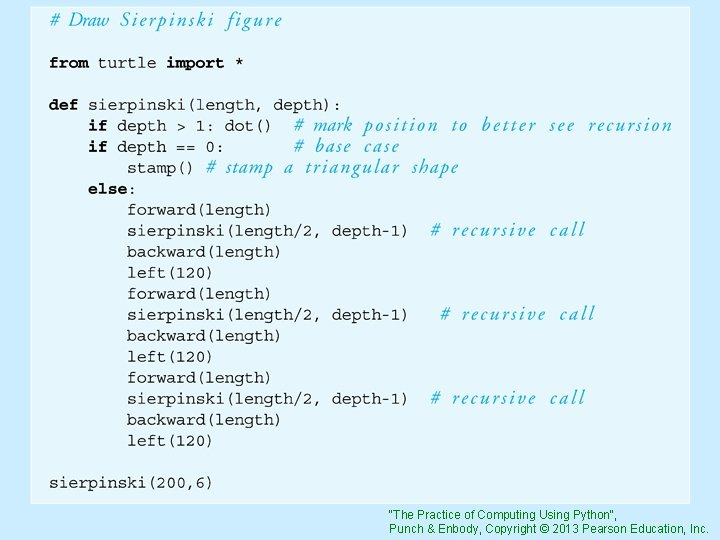"The Practice of Computing Using Python", Punch & Enbody, Copyright © 2013 Pearson Education, Inc.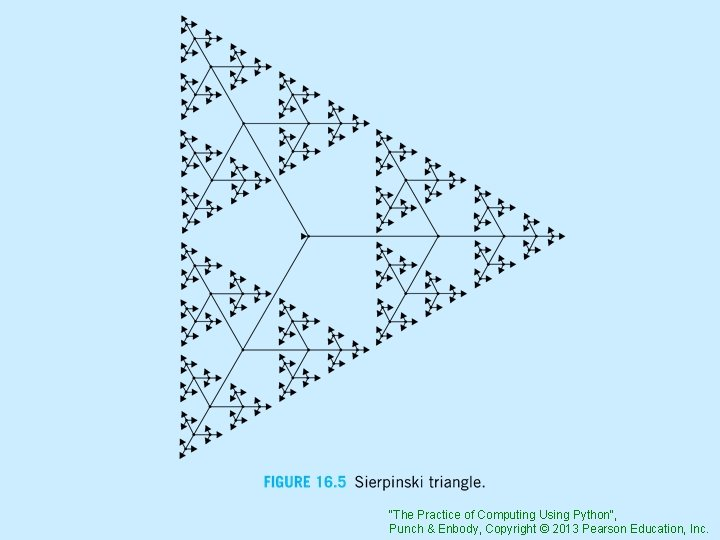"The Practice of Computing Using Python", Punch & Enbody, Copyright © 2013 Pearson Education, Inc.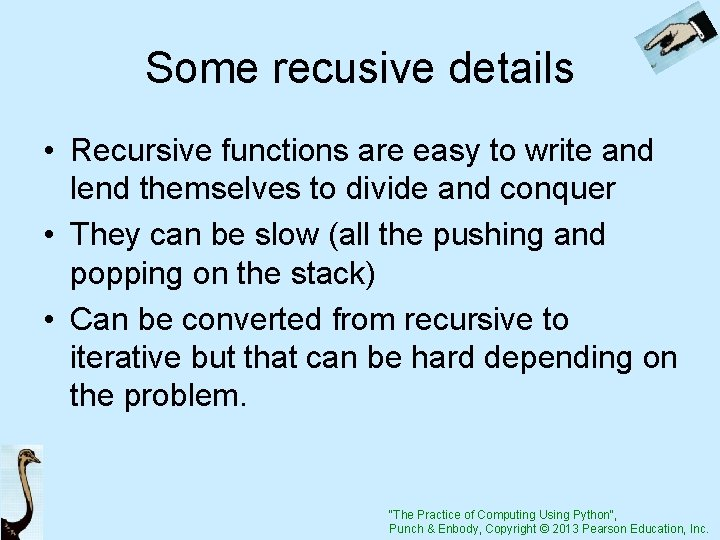Some recusive details • Recursive functions are easy to write and lend themselves to divide and conquer • They can be slow (all the pushing and popping on the stack) • Can be converted from recursive to iterative but that can be hard depending on the problem. "The Practice of Computing Using Python", Punch & Enbody, Copyright © 2013 Pearson Education, Inc.